###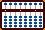PERCENTAGES

In mathematics, a percentage is a way of expressing a number as a fraction of 100. For example:

3% (3 percent) is equal to  3 /100  which is equal to  0.03
27% (27 percent) is equal to  27 /100  which is equal to  0.27
138% (138 percent) is equal to  138 /100  which is equal to  1.38

For those familiar with Japanese soroban techniques for doing multiplication finding the percentage of a number presents no real problems. The fun really begins when we try adding a percentage to a number. I'll illustrate both techniques.

### ▪ Finding a Percentage of a Number

#### Please note; the following two examples use Fukutaro Kato's method to predetermine the unit rod.

Example 1: Find 7% of 23

For abacus work the problem is best expressed as   23 x 0.07 = 1.61

Step1: Rod G will be the unit rod. Using Fukutaro Kato's method set 23 onto rods FG and 7 on rod C. (Fig.1)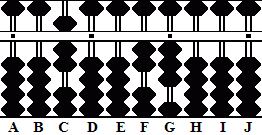Fig.1 ```Step 1 A B C D E F G H I J . . .   . 0 0 7 0 0 2 3 0 0 0 Step 1 ```

Step 2: Multiply 3 on G by 7 and add the product 21 to rods HI. Clear 3 from rod G.

Step 2a & the answer: Multiply 2 on F by 7 and add the product 14 to rods GH. Clear 2 from rod F leaving the answer 1.61 on rods GHI. (Fig.2)Fig.2 ```Steps 2 & 2a A B C D E F G H I J . . .   . 0 0 7 0 0 2 3 0 0 0 + 2 1 clear (-3)      Step 2 0 0 7 0 0 2 0 2 1 0 + 1 4 clear (-2)       Step 2a 0 0 7 0 0 0 1 6 1 0 ```

Example 2: Find 25% of 57

For abacus work the problem is best expressed as   57  x 0.25 = 14.25

Step1: Rod G will be the unit rod. Using Fukutaro Kato's method set 57 on rods EF and 25 on rods AB. (Fig.3)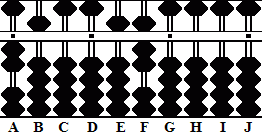Fig.3 ```Step 1 A B C D E F G H I J . . .   . 2 5 0 0 5 7 0 0 0 0 Step 1 ```

Step 2: Multiply 7 on F by 2 on A and add the product 14 to rods GH.

2a: Multiply 7 on F by 5 on B and add the product 35 to rods HI. Clear 7 on F. (Fig.4)Fig.4 ```Steps 2 & 2a A B C D E F G H I J . . .   . 2 5 0 0 5 7 0 0 0 0 + 1 4 Step 2 + 3 5 Step 2a clear (-7)       2 5 0 0 5 0 1 7 5 0 ```

Step 3: Multiply 5 on E by 2 on A and add the product 10 to rods GH.

3a & the answer: Multiply 5 on E by 5 on B and add the product 25 to rods GH. Clear 5 on E leaving the answer 14.25 on rods FGHI. (Fig.5)Fig.5 ```Steps 3 & 3a A B C D E F G H I J . . .   . 2 5 0 0 5 0 1 7 5 0 + 1 0 Step 3 + 2 5 Step 3a clear (-5)       2 5 0 0 0 1 4 2 5 0 ```

### ▪ Adding a Percentage to a Number

While finding the percentage of a number involves simple multiplication, adding a percentage involves a little more agility. Adding a percent requires us to multiply and add the product directly to the original number. Depending on the problem and how many carries it involves this can be awkward because the numbers we're multiplying can change. In other words sometimes we have to keep the original number in our memory while working on the solution. I'll offer three examples; each progressively more difficult.

This first example is straight forward and will provide little to no problem in coming to the solution.

Example 3: Add 7% to 23

##### Note: While I could simply multiply 1.07 x 23 to come up with the same answer, it is slightly more tedious. I've chosen to save a step and omit multiplying by the 1.00 part of the problem and get right to adding the product of   0.07 x 23   to 23 that's already on the frame.  It's really just a matter of knowing which rods to use to come up with a correct answer.

Step1: Rod G will be the unit rod. Set 23 onto rods FG and 7 on rod C. (Fig.6)Fig.6 ```Step 1 A B C D E F G H I J . . .   . 0 0 7 0 0 2 3 0 0 0 Step 1 ```

Step 2: Multiply 3 on G by 7 and add the product 21 to rods HI.

2a & the answer: Multiply 2 on F by 7 and add the product 14 to rods GH leaving the answer 24.61 on rods FGHI. (Fig.7)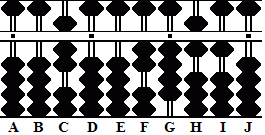Fig.7 ```Steps 2 & 2a A B C D E F G H I J . . .   . 0 0 7 0 0 2 3 0 0 0 + 2 1 Step 2 0 0 7 0 0 2 3 2 1 0 + 1 4 Step 2a 0 0 7 0 0 2 4 6 1 0 ```
##### Notice the technique. In the examples below the order of operation will change slightly from the norm. Rather than starting from the left-hand most number in the multiplier, I find it easier to begin the multiplication using the right-hand most number. While this is not written in stone, I find it less confusing (see step 2 for clarification).

This example requires a little more agility than the previous one.

Example 4: Add 25% to 67

Step1: Rod G will be the unit rod. Set 67 on rods FG and 25 on rods BC. (Fig.8)Fig.8 ```Step 1 A B C D E F G H I J . . .   . 0 2 5 0 0 6 7 0 0 0 Step 1 ```

Step 2: Begin by multiplying 7 on G  by 5 on C (right-hand most number in the multiplier) and add the product 35 to rods HI.

2a: Multiply 7 by 2 on B and add the product 14 to rods GH leaving the interim answer 68.75 on rods FGHI. (Fig.9)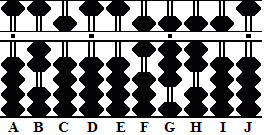Fig.9 ```Steps 2 & 2a A B C D E F G H I J . . .   . 0 2 5 0 0 6 7 0 0 0 + 3 5 Step 2 + 1 4 Step 2a 0 2 5 0 0 6 8 7 5 0 ```

Step 3: Multiply 6 on F by 5 on C and add the product 30 to rods GH.

##### (Note: In this last operation notice how the 6 on F has been bumped up to 7. In this next step remember to multiply by the original 6 on rod F.)

3a & the answer: Keeping 6 in mind multiply it by 2 on B and add the product 12 to rods FG leaving the answer 83.75 on rods FGHI. (Fig.10)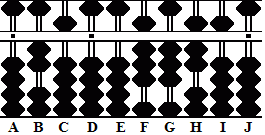Fig.10 ```Steps 3 & 3a A B C D E F G H I J . . .   . 0 2 5 0 0 6 8 7 5 0 + 3 0 Step 3 0 2 5 0 0 7 1 2 5 0 (Interim answer) x 6 + 1 2 Step 3a 0 2 5 0 0 8 3 7 5 0 ```

Example 5: Add 75% to 87

Step1: Rod G will be the unit rod. Set 87 on rods EF and 75 on rods BC. (Fig.11)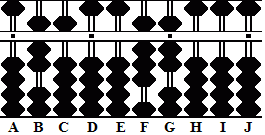Fig.11 ```Step 1 0 A B C D E F G H I J . . .   . 0 7 5 0 0 8 7 0 0 00 Step 1 ```

Step 2: Begin by multiplying  7 on G by 5 on C (right-hand most number in the multiplier) and add the product 35 to rods HI.

2a: Multiply 7 by 7 on B and add the product 49 to rods GH leaving the interim answer 92.25.75 on rods FGHI. (Fig.12)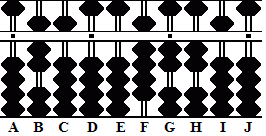Fig.12 ```Steps 2 & 2a A B C D E F G H I J . . .   . 0 7 5 0 0 8 7 0 0 0 + 3 5 Step 2 + 4 9 Step 2a 0 7 5 0 0 9 2 2 5 0 ```
##### (Note: In these next steps remember to multiply by the original 8 on rod F.)

Step 3: Multiply 8 by 5 on C and add the product 40 to rods GH.

3a & the answer: Multiply 8 by 7 on B and add the product 56 to rods FG leaving the answer 152.25 on rods EFGHI. (Fig.13)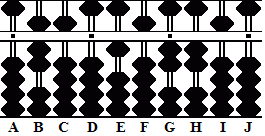Fig.13 ```Steps 3 & 3a A B C D E F G H I J . . .   . 0 7 5 0 0 9 2 2 5 0 x 8 + 4 0 Step 3 2 7 0 0 0 9 6 2 5 0 (Interim answer) x 8 + 5 6 Step 3a 0 7 5 0 1 5 2 2 5 0 ```

Here's a link to some ideas for adding 15% to a number. It's a copy of a message I posted to the Soroban/Abacus Yahoo newsgroup back in in August of 2003.